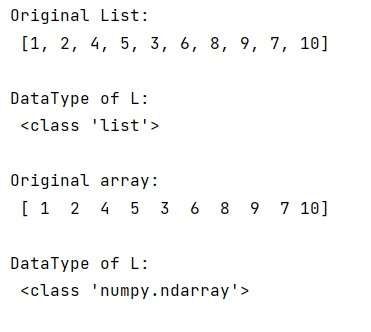# How to save a list as NumPy array?

Learn, how to save a list as NumPy array in Python?
Submitted by Pranit Sharma, on January 02, 2023

NumPy is an abbreviated form of Numerical Python. It is used for different types of scientific operations in python. Numpy is a vast library in python which is used for almost every kind of scientific or mathematical operation. It is itself an array which is a collection of various methods and functions for processing the arrays.

## Saving a list as NumPy array

A list is a collection of heterogeneous elements and it is mutable. Elements inside the list are encapsulated in square brackets. A list is considered a series or collection and we can perform operations like insert, update, and delete with its elements. Series in pandas contains a single list that can store heterogeneous types of data, because of this, a series is also considered a 1-dimensional data structure.

Here, we are going to save or convert a list into a NumPy array. For this purpose, we will first import the array object from the NumPy library and then we will use this object and pass our list inside it as an argument.

Let us understand with the help of an example,

## Python code to save a list as NumPy array

```# Import numpy
import numpy as np

# Import array
from numpy import array

# Creating a list
l = [1,2,4,5,3,6,8,9,7,10]

# Display list
print("Original List:\n",l,"\n")

# Check its data type
print("DataType of L:\n",type(l),"\n")

# Converting the list into array
arr = array(l)

# Display original array
print("Original array:\n",arr,"\n")

# Check its data type
print("DataType of L:\n",type(arr),"\n")
```

Output: